# Division table

## Table of dividing integers 2, 3, 4, 5, 6, 7, 8, 9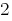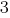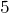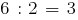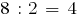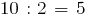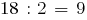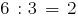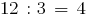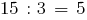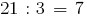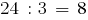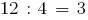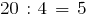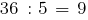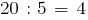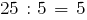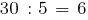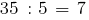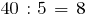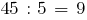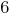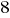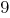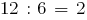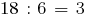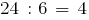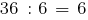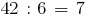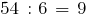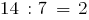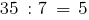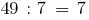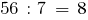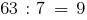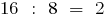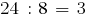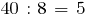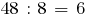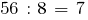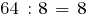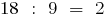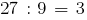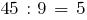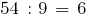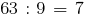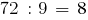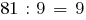### Division table 2

0 : 2=0 (0 divided by 2 turns 0)

### Division table for 3

3 : 3=1 (3 divided by 3, it turns out 1)

### Division table for 4

4 : 4=1 (4 divided by 4, we get 1)

### Division table for 5

25 : 5=5 (25 divided by 5 turns 5)

### Division table for 6

18 : 6=3 (18 divided by 6, giving 3)

### Division table for 7

21 : 7=3 (21 divided by 7, becomes 3)

### Division table for 8

64 : 8=8 (64 divided by 8, it turns out 8)

### Division table for 9

27 : 9=3 (27 divided by 9, gives 3)

Versions in other languages:
Share with friends: